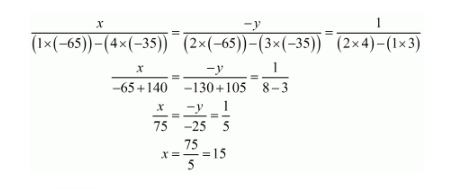# Solve each of the following systems of equations by the method of cross-multiplication :

Question:

Solve each of the following systems of equations by the method of cross-multiplication :

$2 x+y=35$

$3 x+4 y=65$

Solution:

GIVEN:

$2 x+y=35$

$3 x+4 y=65$

To find: The solution of the systems of equation by the method of cross-multiplication:

Here we have the pair of simultaneous equation

$2 x+y-35=0$

$3 x+4 y-65=0$

By cross multiplication method we getAlso $\mathrm{y}=\frac{25}{5}$

Hence we get the value of $x=15$ and $y=5$